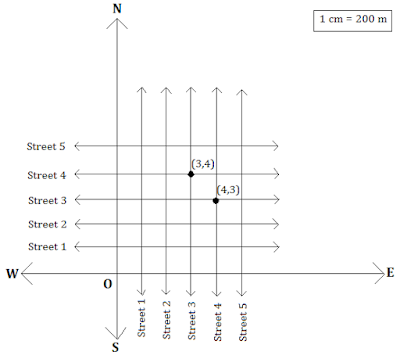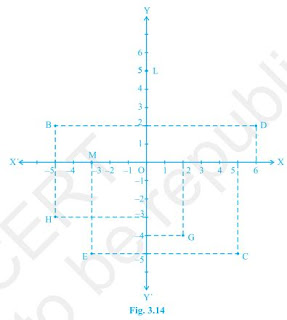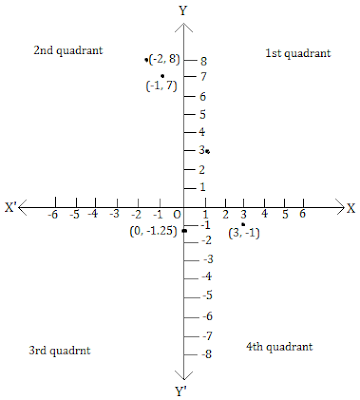>

# NCERT Solutions for Class 9 Maths Chapter 3 Coordinate Geometry| PDF Download

On this page, you will get Chapter 3 Coordinate Geometry Class 9 Maths NCERT Solutions which are accurate and detailed and as per the latest syllabus released by CBSE. You can also Download PDF of Class 9 Maths Chapter 3 Coordinate Geometry NCERT Solutions which will develop you understanding of the chapter and help in gaining good marks in the examinations.

You can also complete your homework on time through the help of these NCERT Solutions and able to solve the difficult problems in a exercise. A student will learn how to solve a questions efficiently and obtain maximum marks in the exams. These NCERT Solutions for Class 9 will prove useful guide in making a student confident.Page No: 53

Exercise 3.1

1. How will you describe the position of a table lamp on your study table to another person?

To describe the position of a table lamp on the study table, we have two take two lines, a perpendicular and horizontal. Considering the table as a plane and taking perpendicular line as Y axis and horizontal as X axis. Take one corner of table as origin where both X and Y axes intersect each other. Now, the length of table is Y axis and breadth is X axis. From The origin, join the line to the lamp and mark a point. Calculate the distance of this point from both X and Y axes and then write it in terms of coordinates.
Let the distance of point from X axis is x and from Y axis is y then the the position of the table lamp in terms of coordinates is (x,y).

2. (Street Plan) : A city has two main roads which cross each other at the centre of the city. These two roads are along the North-South direction and East-West direction.
All the other streets of the city run parallel to these roads and are 200 m apart. There are 5 streets in each direction. Using 1cm = 200 m, draw a model of the city on your notebook. Represent the roads/streets by single lines. There are many cross- streets in your model. A particular cross-street is made by two streets, one running in the North - South direction and another in the East - West direction. Each cross street is referred to in the following manner : If the 2nd street running in the North - South direction and 5 th in the East - West direction meet at some crossing, then we will call this cross-street (2, 5). Using this convention, find:
(i) how many cross - streets can be referred to as (4, 3).
(ii) how many cross - streets can be referred to as (3, 4)(i) Only one street can be referred to as (4, 3) as we see from the figure.
(ii) Only one street can be referred to as (3, 4) as we see from the figure.

Page No: 60

Exercise 3.2

1. Write the answer of each of the following questions:
(i) What is the name of horizontal and the vertical lines drawn to determine the position of any point in the Cartesian plane?
(ii) What is the name of each part of the plane formed by these two lines?
(iii) Write the name of the point where these two lines intersect.

(i) The name of horizontal lines and vertical lines drawn to determine the position of any point in the Cartesian plane is x-axis and y-axis respectively.
(ii) The name of each part of the plane formed by these two lines x-axis and y-axis is quadrants.
(iii) The point where these two lines intersect is called origin.

2. See Fig.3.14, and write the following:
(i) The coordinates of B.
(ii) The coordinates of C.
(iii) The point identified by the coordinates (-3, -5).
(iv) The point identified by the coordinates (2, -4).
(v) The abscissa of the point D.
(vi) The ordinate of the point H.
(vii)The coordinates of the point L.
(viii) The coordinates of the point M.(i) The coordinates of B is (-5, 2).

(ii) The coordinates of C is (5, -5).

(iii) The point identified by the coordinates (-3, -5) is E.

(iv) The point identified by the coordinates (2, -4) is G.

(v) Abscissa means x coordinate of point D. So, abscissa of the point D is 6.

(vi) Ordinate means y coordinate of point H. So, ordinate of point H is -3.

(vii) The coordinates of the point L is (0, 5).

(viii) The coordinates of the point M is (- 3, 0).

Page No: 65

Exercise 3.3

1. In which quadrant or on which axis do each of the points (-2, 4), (3, -1), (-1, 0), (1, 2) and (-3, -5) lie? Verify your answer by locating them on the Cartesian plane.2. Plot the points (x, y) given in the following table on the plane, choosing suitable units of distance on the axes.
 x -2 -1 0 1 3 y 8 7 -1.25 3 -1

Points (x,y) on the plane. 1unit = 1 cm## NCERT Solutions for Class 9 Maths Chapter 3 Coordinate Geometry

Chapter 3 Coordinate Geometry Class 9 Maths NCERT Solutions deals with some basic concepts of coordinate geometry. The system used for describing the position of a point in a plane is also known as the Cartesian system. The mutually perpendicular lines are called axes. The horizontal line is called the x-axis and the vertical line is called the y-axis. The x-coordinate of a point is called the abscissa. The y-coordinate of a point is called the ordinate.

• Cartesian System: The coordinates of the origin O? It has zero distance from both the axes so that its abscissa and ordinate are both zero. Therefore, the coordinates of the origin are (0, 0).You will learn to find a coordinates given a Cartesian plane.

• Plotting a Point in the Plane if its Coordinates are Given: If x ≠ y, then the position of (x, y) in the Cartesian plane is different from the position of (y, x). So, if we interchange the coordinates x and y, the position of (y, x) will differ from the position of (x, y). This means that the order of x and y is important in (x, y). Therefore, (x, y) is called an ordered pair. The ordered pair (x, y) ≠ ordered pair (y, x), if x ≠ y. Also (x, y) = (y, x), if x = y. You will learn to plot the points on a cartesian plane.

There are only three exercises in Coordinate Geometry Class 9 Maths Textbook. If you're facing any type of difficulty while finding a given question then you can look at the exerciseiwse NCERT Solutions given below.

Studyrankers experts have taken every while preparing these Chapter 3 Coordinate Geometry NCERT Solutions and detailed every step so if you find any doubt while solving a question then you can always look from here and move further.

### NCERT Solutions for Class 9 Maths Chapters:

 Chapter 1 Number Systems Chapter 2 Polynomials Chapter 4 Linear Equations in Two Variables Chapter 5 Introduction to Euclid's Geometry Chapter 6 Lines and Angles Chapter 7 Triangles Chapter 8 Quadrilaterals Chapter 9 Areas of Parallelograms and Triangles Chapter 10 Circles Chapter 11 Constructions Chapter 12 Heron's Formula Chapter 13 Surface Areas And Volumes Chapter 14 Statistics Chapter 15 Probability

FAQ on Chapter 3 Coordinate Geometry

#### What are the benefits of NCERT Solutions for Chapter 3 Coordinate Geometry Class 9 NCERT Solutions?

Chapter 3 Coordinate Geometry Class 9 Maths NCERT Solutions will be very useful in revising the chapter thoroughly and understand all the basic concepts. Through the help of these, you will get to know about various terms such as Cartesian plane, quadrants, origin and many others.

#### How to plot a point in the plane when coordinates of the point are given?

To plot a point in the coordinate plane we draw the coordinate axes and choose our units such that we can mark equal distances on the x-axis or on the y-axis or on both the axes. We take origin as zero for x-axis as well as on y-axis. The distances marked along OX and OY are taken as positive and those along OX' and OY' are taken as negative.

#### What are the coordinates of origin?

(0, 0) are the coordinates of origin.

#### What is the sign of the y-coordinate of a point lying in the III-quadrant?

The y-coordinate of a point in III-quadrant is always negative.